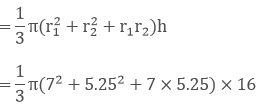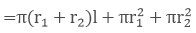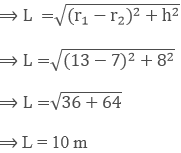or# RD Sharma Class 10 and Chapter Surface Areas And Volumes Maths Solutions

## Maths Class 10 and Chapter Surface Areas And Volumes RD Sharma SolutionsFilter
Filters :
CLASSES
•
•
•
•
•
•
•
CHAPTERS
•
• 6 More

### RD SHARMA Class 10 | SURFACE AREAS AND VOLUMES

A solid sphere of radius 10.5 cm is melted and recast into smaller solid cones, each of radius 3.5cm and height 3cm. Find the number of cones so formed. `(U s epi=(22)/7)`### RD SHARMA Class 10 | SURFACE AREAS AND VOLUMES

From a rectangular sheet of paper ABCD with AB=40CM and AD=28cm, a semi circular portion with BC as diameter is cut off. Find the area of the remaining paper. `(U s epi=(22)/7)`### RD SHARMA Class 10 | SURFACE AREAS AND VOLUMES

In the given figure, a triangle `PQR` is drawn to circumscribe a circle of radius `sqrt(3 )cm` such that segments RS and QS into which QR is divided by the point of contact S are of lengths `5 cm` and `3 cm` respectively. If the area of `DeltaPQR` is `10sqrt(3 )cm^2`, then find the sides PQ and PR.In our previous chapter, we have learned about Areas related to Circles.  Here, in this chapter, we will focus on Surface Areas and Volumes. We will get the best idea on how to calculate the length of different solid cylinders. Students would also be able to get the perfect knowledge on how to calculate the volume, slant surfaces, etc. It has been possible for the students to get the best technique on how to attempt different techniques with the help of Doubtnut.

NCERT Solutions are not so useful for the students to get in-depth knowledge of the subject at all.  For this, it becomes quite problematic for the students to get the perfect idea on how to attempt the trickiest questions that are set in the exams. In order to get rid of their queries and to excel in exams, they opt for the different guides or reference books. Here, RD Sharma Solutions for class 10 maths proves to be quite handy for them.

A team of experienced tutors has come up with the most useful online study materials for aspiring students. With the use of step by step solutions, it becomes much easier for them to get the best understanding of how to attempt different questions in the easiest manner. One can also look forward to downloading the entire study materials in pdf format. So, it becomes quite flexible to access it from anywhere. So, students can get all the perfect information on how to clear their queries in the best way.

## Topics and subtopics of RD Sharma class 10 Chapter 16 Surface Areas and Volumes

### Calculating the length of a solid cylinder

In order to find the length of a solid cylinder, it is important to calculate in an accurate manner. Here, we will have a look at some of the exercises.

Exercise 1: What length of a solid cylinder 2 cm in diameter should be taken to recast into a hollow cylinder of length 16 cm, external diameter 20 cm and thickness 2.5 mm?

Solution:

According to the question

Diameter of the solid cylinder = 2 cm

The recasting of the solid cylinder into a hollow cylinder of length 16 cm, external diameter

of 20 cm and a thickness of 2.5 cm

Volume of the cylinder = πr2h

Radius of the cylinder = 1 cm

So, the volume of the solid cylinder = π12h

Let the length of the solid cylinder be I

Volume of the hollow cylinder = πh (R2- r2).

Thickness of the cylinder = (R - r)

0.25 = 10 - r

Internal radius of the cylinder is 9.75 cm

Volume of the hollow cylinder = π × 16 (100 – 95.0625)

Therefore, we can find that the volume of the solid cylinder is equal to the volume of the hollow cylinder

Equation I = equation ii

π12h = π × 16(100 - 95.06)

h = 79.04 cm

Length of the solid cylinder is 79.04 cm.

Exercise 2: A cylindrical vessel has a diameter equal to its height. It is full of water which is poured into two identical cylindrical vessels with the diameter 42 cm and height 21 cm. Find the diameter of the cylindrical vessel?

Solution:

Given,

The diameter is equal to the height of a cylinder = πr2h

So, volume = πr22r (h = 2r)  ..... (i)

= 2πr2

Volume of each vessel = πr2h

Diameter = 42 cm

Height = 21 cm

Diameter = 2r

2r = 42 cm

r = 21 cm

Volume of vessel = π 212 × 21 ….. (ii)

Here, we find that the volumes of the equation i and ii are equal

So equating both the equations

r3 = (21)3

r = 21 cm

d = 42 cm

Therefore the diameter of the cylindrical vessel is 42 cm.

Exercise 3:  Rainwater which falls on a flat rectangular surface of length 6 m and breadth 4 m is transferred into a cylindrical vessel of internal radius 20 cm. Can you find out what will be the height of water in the cylindrical vessel when a rainfall of 1 cm has fallen?

Solution:

Given,

Here, the length of the rectangular surface = 6 m = 600 cm

Now, the breadth of the rectangular surface = 4 m = 400 cm

Height of the perceived rain = 1 cm

Volume of the rectangular surface = length * breadth * height

= 600*400*1 cm3

= 240000 cm3  …………….. (i)

Given,

Radius of the cylindrical vessel = 20 cm

Let the height of the cylindrical vessel = h cm

Volume of the cylindrical vessel = π × r2 × h

= π × 202 × h         ….. (ii)

Since rains are transferred to cylindrical vessel

So, equating both the above-marked equations:

240000 = π × 202 × h

H = 190.9 cm

Therefore, we find that the height of the cylindrical vessel is 190.9 cm.

Exercise 4:  The perimeters of the ends of a frustum of a right circular cone are 44 cm and 33 cm. If the frustum’s height is 16 cm, calculate its volume, the slant surface and the total surface.

Solution:

Let the slant height of frustum right circular cone be LL = 16.1 cm

Slant height of the frustum cone = 20.37 cm

Curved surface area of the frustum cone = π(r1 + r2)l

= π(7 + 5.25)16.1

Curved surface area of the frustum cone = 619.65 cm3

Volume of the frustum cone= 1898.56 cm3

Volume of the cone = 1898.56 cm3

Total surface area of the frustum cone= π (7 + 5.25) × 16.1 + π72 + π5.252

= π (7 + 5.25) × 16.1 + π (72 + 5.252) = 860.27 cm2

Total surface area of the frustum cone = 860.27 cm2

Exercise 5: There is a tent that consists of a frustum of a cone capped by a cone. If radii of ends of frustum be 13 m and 7 m the height of frustum be 8 m and the slant height of the conical cap be 12 m. Find canvas required for the tent.

Solution:

Given height of frustum (h) = 8 m

We find that the radii of the frustum cone are 13 cm and 7 cm

r1 = 13 m; r2 = 7

Let ‘L’ be slant height of the frustum coneCurved surface area of frustum (s1) = π(r1 + r2) × L= π(13 + 7) × 10 = 200 π m2

Curved surface area of frustum (s1)= 200 π m2

Given slant height of conical cap = 12 m

Here, the base radius of upper cap cone = 7 m

Curved surface area of upper cap cone (s2) = πrL = π × 7 × 12 = 264 m2

Total number of canvas required for tent (S) = s1 + s2

S = 200π + 264 = 892.57m2

Total canvas = 892.57 m2

## Significance of Doubtnut for the students

Students find it quite easy to understand the different concepts of Geometry. They also feel quite confident for being able to get all their queries cleared. As the study materials are written in a very simple language, it does not lead to any sort of problem in understanding it. Let us have a look at the significance of Doubtnut for the students.

• Makes learning fun and exciting:  Learning becomes quite fun and exciting where students develop interests in the subject. So, Geometry proves to be quite interesting with the help of the video tutorials. So, it proves to be of much use to the students where they are not deprived of any important and useful knowledge.  This also makes them score good marks in the exams without any worry at all.
• Helps to provide good techniques: In Geometry, students should be able to clear all their queries in the best way. The best technique is provided by the team of Doubtnut where the students do not find it difficult at all to attempt even the trickiest questions that are set in the exams. So, it helps a lot to get an accurate idea on how to solve the different questions.
• Lots of exercises and examples included: Students can find lots of exercises and examples that truly helps to practice it in the best way. So, achieving success in the examination becomes possible where students do not have to stay confused for any sort of reasons at all.